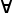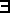# Maths - Logic and Boolean Algebra

Logic is a language for reasoning.

On these pages I am mostly concerned with mathematical logic and the mathematical structures that are related to it. There are a common set of lattice-like structures that occur in various branches of mathematics such as orders, logic and sets.

Order Logic Set
T top true universe
_|_ bottom false Ø
/\ (conjunction) greatest lower bound and\/ (disjunction) least upper bound or U

## Logic

We often write a rule in logic like this:
where A and B are propositions.
and A/\B is an assertion

 A B A/\B

We take this to mean that, if all the things above the line are true, then the thing below the line is true.

#### Constants

Values have two values ether true of false, we may use alternative names as follows:

 true 1 T false 0In boolean algebra every value evaluates to either true or false. In intuitionistic logic or constructive logic a value may be neither true or false, it is only true or false when proven to be true or proven to be false.

#### Connectives

 conjunction /\ and (boolean,boolean) -> boolean disjunction \/ or (boolean,boolean) -> boolean negation ¬ not boolean -> boolean implication ==> if (boolean,boolean) -> boolean equivalence <=> if and only if (iff) (boolean,boolean) -> boolean

#### Quantifiersfor allthere exists (for some)

## Propositional, FOL and HOL

Propositional Logic

Has connectives:

• and
• or
• negate
• implication

Propositions could be seen as questions about set membership, for example,

`x is a member of X`

since we can construct X as a set with the property we want.

First Order Logic

Extends Propositional Logic so it has connectives plus:

• predicates (a function that takes inputs and produces true/false).
• quantifiers(range over sets)

Predicates act like functions that take an input value and produce a true/false value.

Higher Order Logic

As First Order Logic plus:

• metapredicate (can take predicates as input)
• quantifiers which can range over sets of sets.
 Questions about sets of sets and so on. Example: all members of Y are members of X:## Boolean Algebra

A B ¬A ¬B or and xor A ==> B A <=> B
0 0 1 1 0 0 0 1 1
0 1 1 0 1 0 1 1 0
1 0 0 1 1 0 1 0 0
1 1 0 0 1 1 0 1 1

### Laws

identity and or
commutativity x /\ y = y /\ x x \/ y = y \/ x
associativity (x /\ y) /\ z = x /\ (y /\ z) (x \/ y) \/ z = x \/ (y \/ z)
idempotency x /\ x = x x \/ x = x
absoption laws x \/ (x /\ y) = x x /\ (x \/ y) = x
distribution (x \/ y) /\ z = (x /\ z) \/ (y /\ z)
excluded middle x /\ ¬x = false x \/ ¬x = true
De Morgan
(duality principle)
¬(x /\ y) = ¬x \/ ¬y ¬(x \/ y) = ¬x /\ ¬y
double negation ¬¬x = x
true and false

x /\ true = x
x /\ false = false
¬true = false

x \/ true = true
x \/ false = x
¬false = true

### Canonical Form

 minterm form ¬A¬B ƒ(00) + ¬AB ƒ(01) + A¬B ƒ(10) + AB ƒ(11) maxterm form (¬A + ¬B + ƒ(00) )( ¬A + B + ƒ(01) )( A + ¬B + ƒ(10) )( A + B + ƒ(11))

example: exclusive or is:

Σ(1,2)=∏(0,3)

### Karnaugh Maps

A\B   0     1
EF\CD     EF\CD
0
 0 4 12 8 1 5 13 9 3 7 15 11 2 6 14 10

 16 20 28 24 17 21 29 25 19 23 31 27 18 22 30 26

EF\CD     EF\CD
1
 32 36 44 40 33 37 45 41 35 39 47 43 34 38 46 42

 48 52 60 56 49 53 61 57 51 55 63 59 50 54 62 58

## Logic and Computing

There is a deep connection between: λ-calculus, intuitionistic logic and cartesian closed categories.Curry–Howard–Lambek correspondence:

Cartesian Closed Category λ-calculus intuitionistic logic
objects

types
p:P

proposition
p = proposition
P = proof of p
Operator Types

function type
b(a):A->B

implication
A implies B
product type
<a,b>:A/\B
conjunction
if A is proof of 'a'
and B is proof of 'b'
then A/\B is proof of <a,b>
sum type
a+b:A\/B
disjunction
if A is proof of 'a'
and B is proof of 'b'
then A\/B is proof of a+b
Dependant Types
dependent product type
The type of the result B(a) depends on the value a .
universal quantification
(a:A).B(a)

dependent sum type
a of type A meets the specification B(a) as proved by b:B(a)
Can be used to represent abstract data types.

existential quantification
(a:A).B(a)

unit type true formula
T
bottom type false formula
_|_
Inductive Types
recursive function inductive proof

morphisms terms proof
variable axiom
constructor introduction rule
destructor elimination rule
normal form normal deduction
weak normalisation normalisation of deductions
type inhabitation problem provability
inhabited type intuitionistic tautology
function application
substitution

### simply typed λ-calculus

where:

• x,y... (lower case) = variables
• A,B ... (upper case, beginning alphabet) = types
• M,N ... (upper case, middle alphabet) = metavariables for terms

Formation rules for well typed terms (wtt):

• every variable x:A is a wtt.
• if x:A is a variable and M:B is a wtt then λ x:A.M: A->B is a wtt
• if M:A -> B is a wtt and N:A is a wtt then M N: B is a wtt
• if M:A is a wtt and N:B is a wtt then <M,N>: A×B is a wtt
• if M:A×B is a wtt then fst(M): A is a wtt
• if M:A×B is a wtt then snd(M): B is a wtt

Given a wtt M:B the set FV(M) of the free variables of M, is defined as follows:

• if M=x, then FV(M) = {x}
• if M=λ x:A.N, then FV(M) = FV(N)-{x}
• if M=N P, then FV(M) = FV(N) U FV(P)
• if M=<N,P>, then FV(M) = FV(N) U FV(P)
• if M=fst(N), then FV(M) = FV(N)
• if M=snd(N), then FV(M) = FV(N)

The substitution [M/x]N:B of a proof M:A for a generic x:A in a proof N:B is defined in the following way:

• if N:B = x:A then [M/x]N:B = M:A
• if N:B = y:A,x≠y then [M/x]N:B = N:B
• if N:B = λ x:C.P:B then [M/x]N:B = λ x:C.P:B
• if N:B = λ y:C.P:B,x≠y,y∉FV(M) then [M/x]N:B = λ y:C.[M/x]P:B
• if N:B = P Q then [M/x]N:B = [M/x]P[M/x]Q:B
• if N:B = <P,Q> then [M/x]N:B = <[M/x]P[M/x]Q>:B
• if N:B = fst(P) then [M/x]N:B = fst([M/x]P)
• if N:B = snd(P) then [M/x]N:B = snd([M/x]P)

Reduction - rewriting system

• α-reduction: λ x:A.M = λ y:A.[y/x]M
• β(->)-reduction: (λ x:A.M)N = [N/x]M
• η(->)-reduction: λ x:A.(M x) = M, if x∉FV(M)
• β(×)-reduction: fst(<M,N>) = M
• β(×)-reduction: snd(<M,N>) = N
• η(×)-reduction: <fst(P),snd(P)>) = P

where:

= : minimal congruence relation